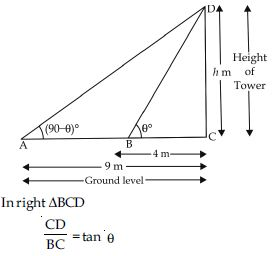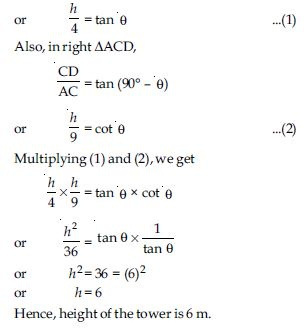# NCERT Solutions for Class 10 Math Chapter 9 - Some Applications of Trigonometry

The NCERT Solutions are prepared by subject matter experts and are available for free for students of Class 10th. They can take the help of these solutions in order to boost their confidence. The entire chapter is accurately covered so as not to leave any concept. Students can rely on the NCERT Solutions for Class 10th to build a strong foundation of the concept included in the chapter “Some Applications of Trigonometry”. The key to scoring good marks in Math is practice. These solutions will polish students’ mathematical skills hence, helping them solve complex questions efficiently.

“Some Applications of Trigonometry” is the continuation of the earlier chapter which is “Introduction to Trigonometry”. In this chapter, students will get to know how to calculate the height and distance of different objects by using trigonometry. Also, they will understand its application in construction, navigation, and determining the position of any land on the basis of its longitudes and latitudes.

##### Question 1:

A circus artist is climbing a 20 m long rope, which is tightly stretched and tied from the top of a vertical pole to the ground. Find the height of the pole, if the angle made by the rope with the ground level is 30$°$ (see fig.).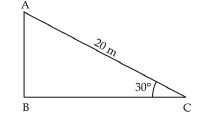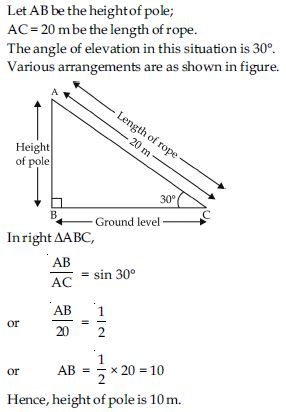##### Question 2:

A tree breaks due to storm and the broken part bends so that the top of the tree touches the ground making an angle 30$°$ with it. The distance between the foot of the tree to the point where the top touches the ground is 8 m. Find the height of the tree. Let BD be length of tree before storm. After storm AD = AC = length of broken part of tree.

The angle of elevation in this situation is 30$°$. Various arrangements are as shown in the figure.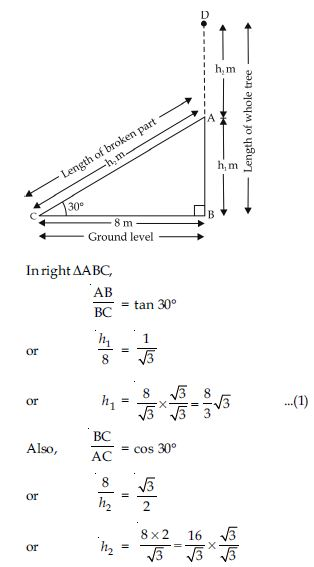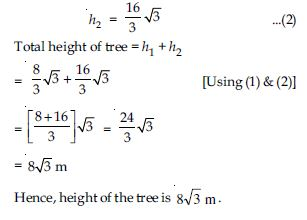##### Question 3:

A contractor plans to install two slides for the children to play in a park. For the children below the age of 5 years, she prefers to have a slide whose top is at a height of 1.5 m, and is inclined at an angle of 30$°$ to the ground, whereas for elder children, she wants to have a steep slide at a height of 3 m, and inclined at an angle of 60$°$ to the ground. What should be the length of the slide in each case?

Case I. For children below 5 years.
Let AC = l1 m denote the length of slide and
BC = 1.5 m be the height of slide. The angle of
elevation is 30$°$.
Various arrangements are shown in figure.
In right $∆$ABC,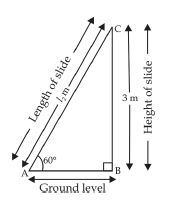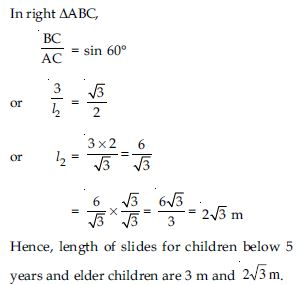##### Question 4:

The angle of elevation of the top of a tower from a point on the ground, which is 30 m away from the foot of the tower, is 30$°$. Find the height of the tower.

Let BC = h m be the height of tower and AB = 30 m be the distance at ground level. Various arrangements are as shown in figure.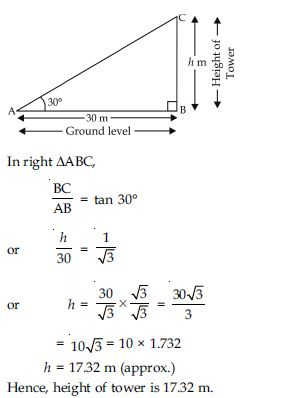##### Question 5:

A kite is flying at a height of 60 m above the ground. The string attached to the kite is temporarily tied to a point on the ground. The inclination of the string with the ground is 60$°$. Find the length of the string, assuming that there is no slack in the string.

Let us suppose position of the kite is at point C and AC = l m be length of string with kite is attached. The angle of elevation for this situation be 60$°$. Various arrangements are as shown in the figure.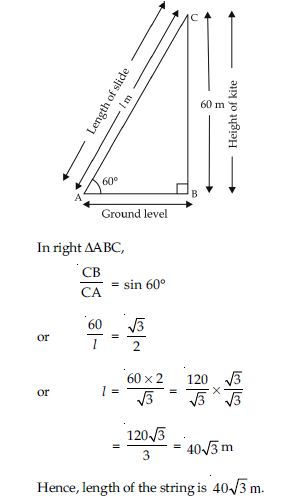##### Question 6:

A 1.5 m tall boy is standing at some distance from a 30 m tall building. The angle of elevation from his eyes to the top of the building increases from 30$°$ to 60$°$ as he walks towards the building. Find the distance he walked towards the building.

Let ED = 30 m be the height of building and EC = 1.5 m be the height of boy. The angle of elevation at different situation are 30$°$ and 60$°$ respectively.

Various arrangements are as shown in figure.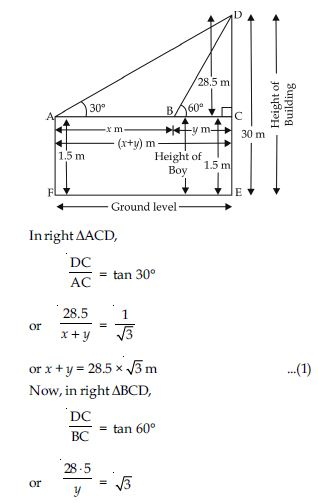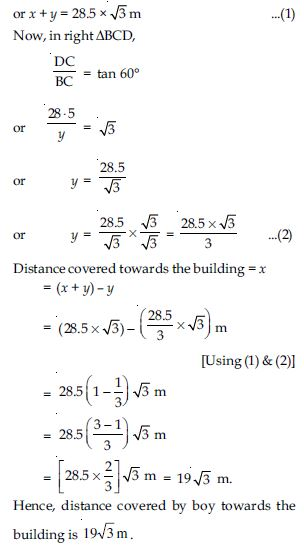##### Question 7:

From a point on the ground, the angles of elevation of the bottom and top of a transmission tower fixed at the top of a 20 m high building are 45$°$ and 60$°$ respectively. Find the height of the tower.

Let BC = 20 m be the height of building and DC = h m be the height of transmission tower. The angle of elevation of the bottom and top of a transmission tower are 45$°$ and 60$°$ respectively. Various arrangements are as shown in the figure.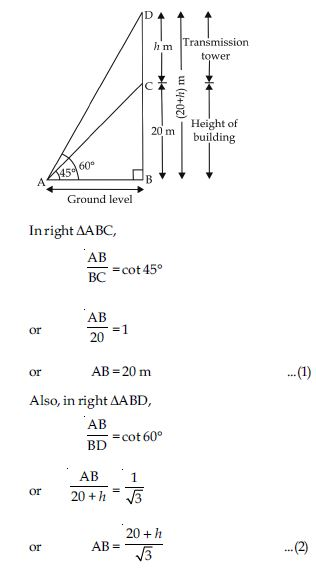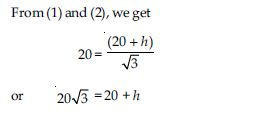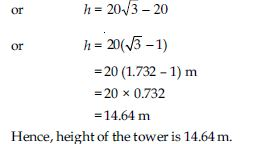##### Question 8:

A statue 1.6 m tall stands on the top of a pedestal. From a point on the ground, the angle of elevation of the top of the statue is 60$°$ and from the same point the angle of elevation of the top of the pedestal is 45$°$. Find the height of the pedestal.

Let BC = h m be the height of pedestal and CD = 1.6 m be the height of statue. The angle of elevation of top of statue and top pedestal are 60$°$ and 45$°$ respectively. Various arrangements are as shown in the figure.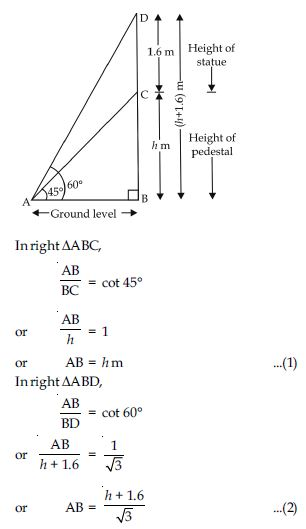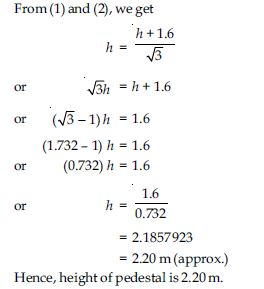##### Question 9:

The angle of elevation of the top of a building from the foot of the tower is 30$°$ and the angle of elevation of the top of the tower from the foot of the building is 60$°$. If the tower is 50 m high, find the height of the building.

Let BC = 50 m be height of tower and AD = h m be height of building. The angle of elevation of the top of a building from the foot of tower and top of tower from foot of the building are 30$°$ and 60$°$ respectively. Various arrangements are as shown in figure.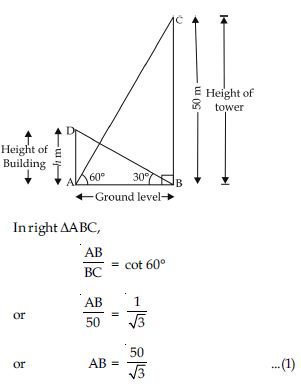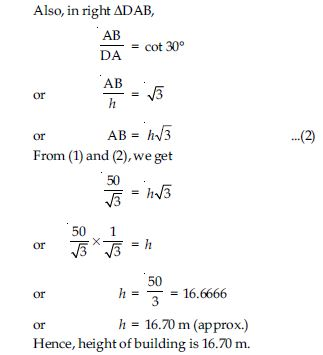##### Question 10:

Two poles of equal heights are standing opposite each other on either side of the road, which is 80 m wide. From a point between them on the road the angles of elevation of the top of the poles are 60$°$ and 30$°$ respectively. Find the height of the poles and the distances of the point from the poles.

Let BC = DE = h m be height of two equal poles and point A be the required position where the angle of elevations of top of two poles are 30$°$ and 60$°$ respectively. Various arrangement are as shown in the figure.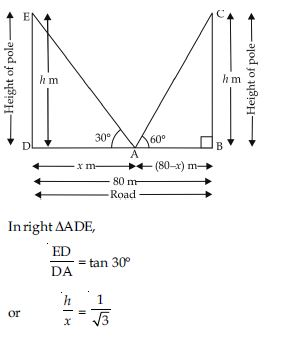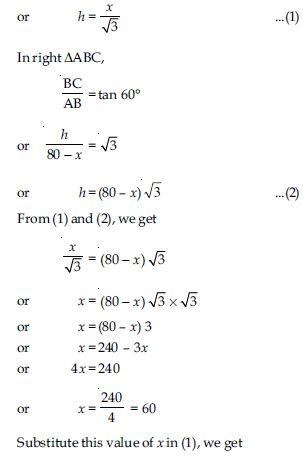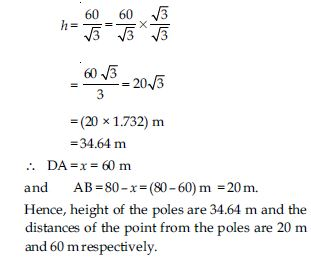##### Question 11:

A TV tower stands vertically on a bank of a canal. From a point on the other bank directly opposite the tower, the angle of elevation of the top of the tower is 60$°$. From a point 20 m away from this point on the same bank, the angle of elevation of the top of the tower is 30$°$ (see fig.). Find the height of the tower and the width of the canal.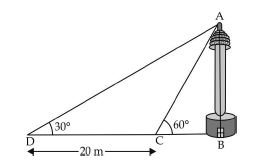Let BC = x m be the width of canal and CD = h m be height of TV tower. The angles of elevation of top of tower at different positions are 30$°$ and 60$°$ respectively. Various arrangements are as shown in figure.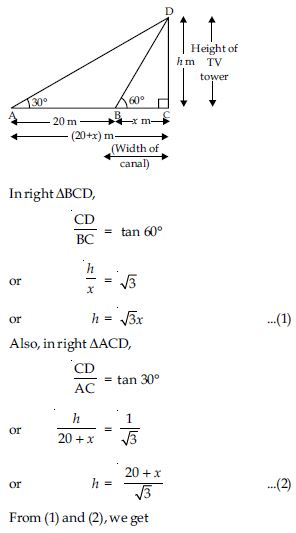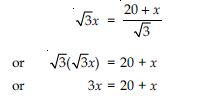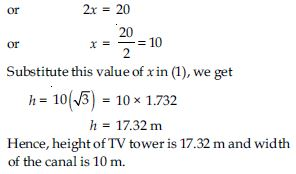##### Question 12:

From the top of a 7 m high building, the angle of elevation of the top of a cable tower is 60$°$ and the angle of depression of its foot is 45$°$. Determine the height of the tower.

Let BD = h m be the height of cable tower and AE = 7 m be the height of building. The angle of elevation of the top of a cable tower and angle of depression of its foot from top of a building are 60$°$ and 45$°$ respectively. Various arrangement are as shown in figure.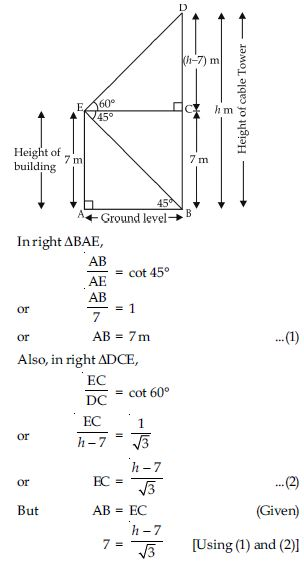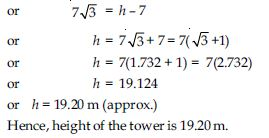##### Question 13:

As observed from the top of a 75 m high lighthouse from the sea-level, the angles of depression of two ships are 30$°$ and 45$°$. If one ship is exactly behind the other on the same side of the lighthouse, find the distance between the two ships.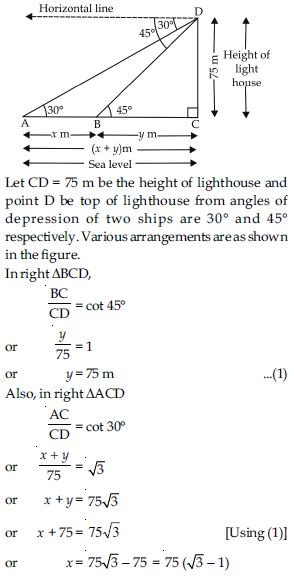or x =54.90
Hence, distance between the two ships is
54.90 m.

##### Question 14:

A 1.2 m tall girl spots a balloon moving with the wind in a horizontal line at a height of 88.2 m from the ground. The angle of elevation of the balloon from the eyes of the girl at any instant is 60$°$. After some time, the angle of elevation reduces to 30$°$ (see fig.). Find the distance travelled by the balloon during the interval.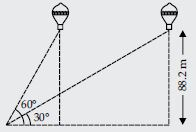Let ‘A’ be the position of 1.2 m tall girl, at the point of the angles of elevation of balloon at different distances are 30$°$ and 60$°$ respectively. Also, BE = CD = 88.2 m be height of balloon. Various arrangements are as shown in the figure.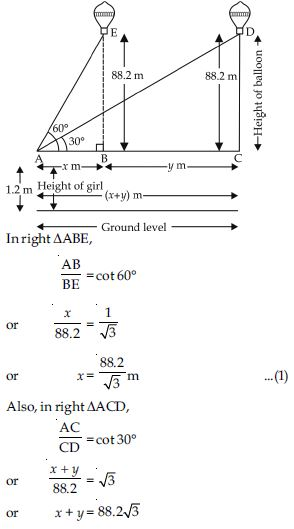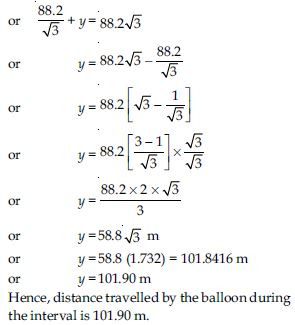##### Question 15:

A straight highway leads to the foot of a tower. A man standing at the top of the tower observes a car at an angle of depression of 30$°$, which is approaching the foot of the tower with a uniform speed. Six seconds later, the angle of depression of the car is found to be 60$°$. Find the further time taken by the car to reach the foot of the tower from this point.

Let CD be the tower of height h metres. Let A be initial position of the car and after six seconds the car be at B. The angles of depression at A and B are 30$°$ and 60$°$ respectively. Various arrangements are as shown in figure.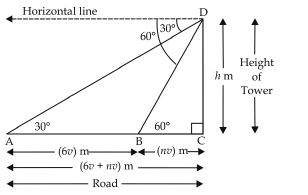Let speed of the car be v metre per second, using formula, Distance = Speed × Time AB = Distance covered by car in 6 seconds AB = 6v metre Also, time taken by car to reach the tower be ‘n’ seconds.
∴ BC = nv metre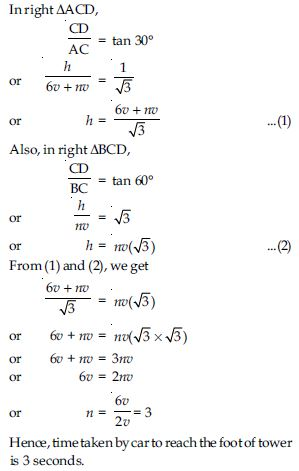##### Question 16:

The angles of elevation of the top of a tower from two points at a distance of 4 m and 9 m from the base of the tower and in the same straight line with it are complementary. Prove that the height of the tower is 6 m.